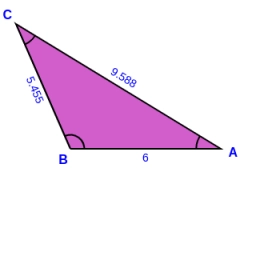# Calculate 9701

In the triangle, the side length AB = 6 cm, the height per side c = 5 cm, the angle BCA = 35°. Calculate sides a, b.

a =  5.455 cm
b =  9.5881 cm

### Step-by-step explanation:

Try calculation via our triangle calculator.Did you find an error or inaccuracy? Feel free to write us. Thank you!

Tips for related online calculators
Do you have a linear equation or system of equations and looking for its solution? Or do you have a quadratic equation?
Do you want to convert length units?
Do you want to convert time units like minutes to seconds?

#### Grade of the word problem:

We encourage you to watch this tutorial video on this math problem: